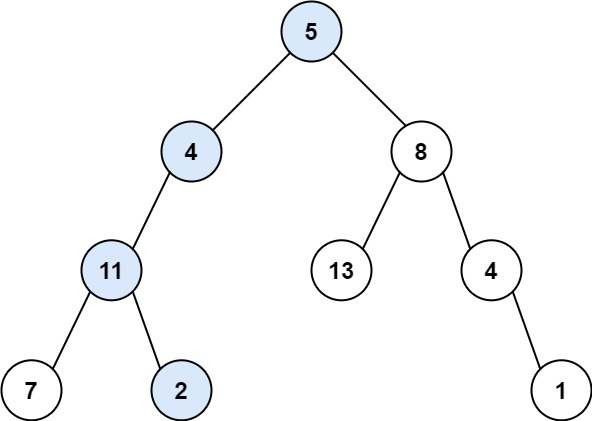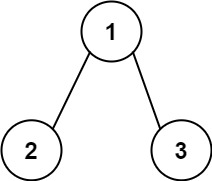## 112. Path Sum

Given the `root` of a binary tree and an integer `targetSum`, return `true` if the tree has a root-to-leaf path such that adding up all the values along the path equals `targetSum`.

A leaf is a node with no children.

Example 1:```Input: root = [5,4,8,11,null,13,4,7,2,null,null,null,1], targetSum = 22
Output: true
```

Example 2:```Input: root = [1,2,3], targetSum = 5
Output: false
```

Example 3:

```Input: root = [1,2], targetSum = 0
Output: false
```

Constraints:

• The number of nodes in the tree is in the range `[0, 5000]`.
• `-1000 <= Node.val <= 1000`
• `-1000 <= targetSum <= 1000`

## Rust Solution

``````struct Solution;
use rustgym_util::*;

trait PathSum {
fn has_path_sum(&self, sum: i32) -> bool;
}

fn has_path_sum(&self, sum: i32) -> bool {
if let Some(node) = self {
let node = node.borrow();
let val = node.val;
let left = &node.left;
let right = &node.right;
if left.is_none() && right.is_none() {
sum == val
} else {
right.has_path_sum(sum - val) || left.has_path_sum(sum - val)
}
} else {
false
}
}
}

impl Solution {
fn has_path_sum(root: TreeLink, sum: i32) -> bool {
root.has_path_sum(sum)
}
}

#[test]
fn test() {
let root = tree!(
5,
tree!(4, tree!(11, tree!(7), tree!(2)), None),
tree!(8, tree!(13), tree!(4, None, tree!(1)))
);
let sum = 22;
let res = true;
assert_eq!(Solution::has_path_sum(root, sum), res);
let root = tree!(1, tree!(2), None);
let sum = 1;
let res = false;
assert_eq!(Solution::has_path_sum(root, sum), res);
}
``````

Having problems with this solution? Click here to submit an issue on github.